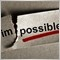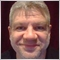# Issue with stoploss and take profit (130 error on ordermodify)876

Hello everyone,

I get an error 130 when I am doing an OrderModify.

The code is:

```void SetSLTP(){
double sl, tp;
for (int i = OrdersTotal() - 1; i >= 0; i--)
if (OrderSymbol() == Symbol() && OrderMagicNumber() == Magic && (OrderType() == OP_BUY || OrderType() == OP_SELL))
if (OrderStopLoss() == 0 && OrderTakeProfit() == 0) {
sl = 0; tp = 0;
tp = profitUp + PipsToPoints(10);
sl = slSecurityDown;
}
else if(OrderType() == OP_SELL){
tp = profitDown - PipsToPoints(10);
sl = slSecurityUp;
}
if (sl != 0 || tp != 0){
_res = OrderModify(OrderTicket(), OrderOpenPrice(), 9598.8, tp, 0);
int err = GetLastError();
if(!_res && err > 0){
Print(MarketInfo(Symbol(),MODE_STOPLEVEL));
Print("SetSLTP: OrderModify(#"+IntegerToString(OrderTicket())+"  @"+DoubleToStr(OrderOpenPrice(), _Digits)
+") - SL("+DoubleToStr(OrderStopLoss(), _Digits)+"): " + DoubleToStr(sl, _Digits) + " TP("+DoubleToStr(OrderStopLoss(), _Digits)+"):" + DoubleToStr(tp, _Digits));
Print("Error in OrderModify. Error code=",err, " -> ", ErrorDescription(err));
Print("");
}
}
}
}```

The vars has values:

```profitUp=9688.8
profitDown=9608.75
slSecurityDown=9588.75
slSecurityUp=9708.75```

The print output is:

```2015.01.08 08:19:43.302 #FDXH5,M1: Error in OrderModify. Error code=130 -> invalid stops
2015.01.08 08:19:43.302 #FDXH5,M1: SetSLTP: OrderModify(#38220494  @9646.0) - SL(0.0): 9708.8 TP(0.0):9598.8
2015.01.08 08:19:43.302 #FDXH5,M1: 20.0
```

I don't know where canbe the error...I have checked everything and no sense!!42596

dream3r:

Hello everyone,

I get an error 130 when I am doing an OrderModify.

The code is:

The vars has values:

The print output is:

I don't know where canbe the error...I have checked everything and no sense!!

Are you trying to set SL at same value as TP ?

`            _res = OrderModify(OrderTicket(), OrderOpenPrice(), 9598.8, tp, 0);`
`2015.01.08 08:19:43.302 #FDXH5,M1: SetSLTP: OrderModify(#38220494  @9646.0) - SL(0.0): 9708.8 TP(0.0):9598.8`876

Yes, sorry. One of my test was wrong and I was just putting here the wrong copy/paste.

The code that does not work is:

```void SetSLTP(){
double sl, tp;
for (int i = OrdersTotal() - 1; i >= 0; i--)
if (OrderSymbol() == Symbol() && OrderMagicNumber() == Magic && (OrderType() == OP_BUY || OrderType() == OP_SELL))
if (OrderStopLoss() == 0 && OrderTakeProfit() == 0) {
sl = 0; tp = 0;
tp = profitUp + PipsToPoints(10);
sl = slSecurityDown;
}
else if(OrderType() == OP_SELL){
tp = profitDown - PipsToPoints(10);
sl = slSecurityUp;
}

if (sl != 0 || tp != 0){
_res = OrderModify(OrderTicket(), OrderOpenPrice(), sl, tp, 0);
int err = GetLastError();
if(!_res && err > 0){
Print(MarketInfo(Symbol(),MODE_STOPLEVEL));
Print("SetSLTP: OrderModify(#"+IntegerToString(OrderTicket())+"  @"+DoubleToStr(OrderOpenPrice(), _Digits)
+") - SL("+DoubleToStr(OrderStopLoss(), _Digits)+"): " + DoubleToStr(sl, _Digits) + " TP("+DoubleToStr(OrderStopLoss(), _Digits)+"):" + DoubleToStr(tp, _Digits));
Print("Error in OrderModify. Error code=",err, " -> ", ErrorDescription(err));
Print("");
}
}
}
}```

And the result it's the same as the first post. I can not understand why. Can you help me please?42596

dream3r:

Yes, sorry. One of my test was wrong and I was just putting here the wrong copy/paste.

The code that does not work is:

And the result it's the same as the first post. I can not understand why. Can you help me please?

Same question as in your other topic. What is current price Bid/Ask at the time you are trying to change SL/TP ?876

Hello,
The bid/ask was so far from this point. In fact, the strange thing is that in demo account, when the EA gives me this error, I tried to put it manually and it works!! So, it makes more strange the thing...

Can you help me to solve the problem please?42596

dream3r:
Hello,
The bid/ask was so far from this point. In fact, the strange thing is that in demo account, when the EA gives me this error, I tried to put it manually and it works!! So, it makes more strange the thing...

Can you help me to solve the problem please?

```_res = OrderModify(OrderTicket(), OrderOpenPrice(), NormalizeDouble(sl,_Digits), NormalizeDouble(tp,_Digits), 0);